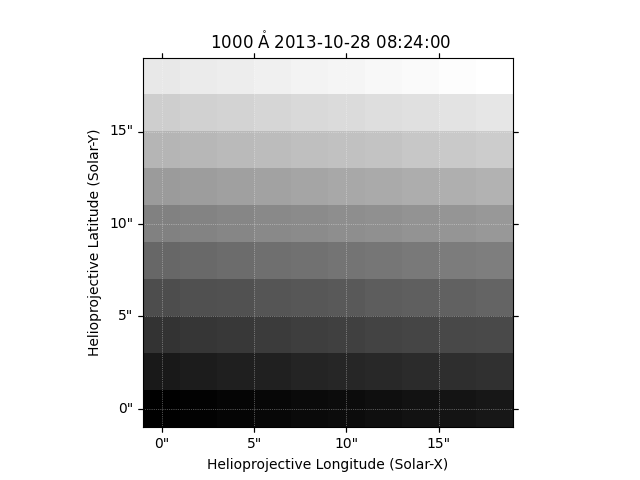# Generating a map from data array#

A simple demonstration of creating a map from a numpy array of data.

import matplotlib.pyplot as plt
import numpy as np

import astropy.units as u
from astropy.coordinates import SkyCoord

import sunpy.map
from sunpy.coordinates import frames


Let’s create some data.

data = np.arange(0, 100).reshape(10, 10)


Next we need to create the metadata. This is made easier using the make_fitswcs_header() function which will create a header object for you. First define the reference coordinate which requires a time and an observer location.

coord = SkyCoord(0*u.arcsec, 0*u.arcsec, obstime='2013-10-28 08:24',
observer='earth', frame=frames.Helioprojective)


Let’s pass that into the helper function along with some parameters. The reference pixel is the pixel is the one at the reference coordinate. The scale sets the size of the pixels. You can also to set a number of other metadata as well such as the instrument name and wavelength.

header = sunpy.map.make_fitswcs_header(data, coord,
reference_pixel=[0, 0]*u.pixel,
scale=[2, 2]*u.arcsec/u.pixel,
telescope='Fake Telescope', instrument='UV detector',
wavelength=1000*u.angstrom)


Let’s now create our map.

manual_map = sunpy.map.Map(data, header)


Let’s plot the result.

fig = plt.figure()